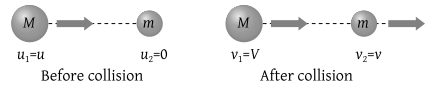A smooth sphere of mass M moving with velocity u directly collides elastically with another sphere of mass m at rest. After collision their final velocities are V and v respectively. The value of v is

(1) $\frac{2uM}{m}$

(2) $\frac{2um}{M}$

(3) $\frac{2u}{1+\frac{m}{M}}$

(4) $\frac{2u}{1+\frac{M}{m}}$

Concept Videos :-

#4 | Definition of Momentum
#5 | Conservation of Momentum
#6 | Problems

Concept Questions :-

Linear momentum

(3)${v}_{2}=\left(\frac{{m}_{2}-{m}_{1}}{{m}_{1}+{m}_{2}}\right)\text{\hspace{0.17em}}{u}_{2}+\frac{2{m}_{1}{u}_{1}}{{m}_{1}+{m}_{2}}=\frac{2Mu}{M+m}=\frac{2u}{1+\frac{m}{M}}$

Difficulty Level:

• 14%
• 23%
• 53%
• 12%